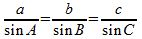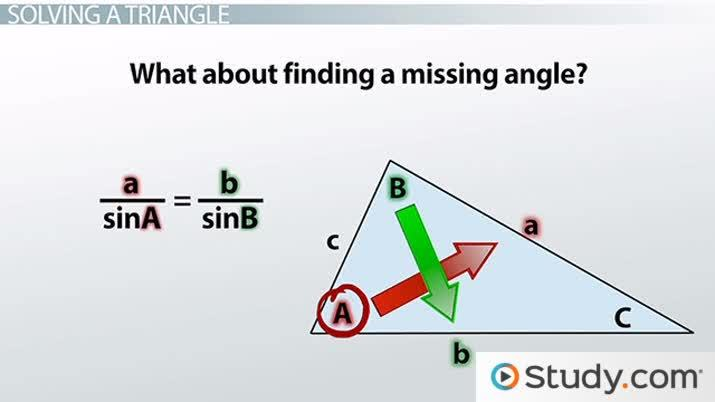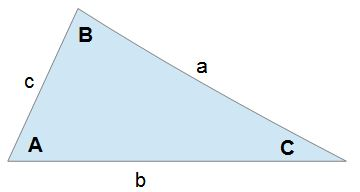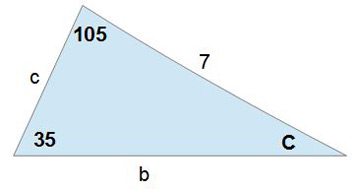# Using the Law of Sines to Solve a Triangle

Lesson Transcript
Instructor: Yuanxin (Amy) Yang Alcocer

Amy has a master's degree in secondary education and has taught math at a public charter high school.

After watching this video lesson, you will be able to use the law of sines to find a missing side of a triangle as well as a missing angle of a triangle. Learn what you need to know about the triangle in order to use the formula.

## Law of Sines

There are many useful formulas in trigonometry. The one we will be talking about in this video lesson is the law of sines, which tells you how the sides and angles of a triangle are related. By using this formula, you can solve any triangle for a missing side or angle. Recall that your basic trig functions of sine, cosine, and tangent can easily be found by using a right triangle. Remember SOHCAHTOA? It tells you that:

• Sine is equal to opposite/hypotenuse
• Cosine is equal to adjacent/hypotenuse
• Tangent is equal to opposite/adjacent

While our basic trig functions are fractions of the various sides of a right triangle, by using our trig functions in a more elaborate way, we can actually use them to solve problems related to any kind of triangle, not just right triangles. This opens up a whole new world of problems that we can solve using trigonometry. Let's take a look at the law of sines. It looks like this:The small lower case letters refer to the sides of a triangle. The larger upper case letters refer to the corresponding angles of the triangle. So, angle A is opposite side a, angle B is opposite side b, and angle C is opposite side c.An error occurred trying to load this video.

Try refreshing the page, or contact customer support.

Coming up next: Solving Real World Problems Using the Law of Sines

### You're on a roll. Keep up the good work!

Replay
Your next lesson will play in 10 seconds
• 0:01 Law of Sines
• 1:22 Solving a Triangle
• 3:28 Example 1
• 4:38 Example 2
• 5:40 Lesson Summary
Save Save

Want to watch this again later?

Timeline
Autoplay
Autoplay
Speed Speed## Solving a Triangle

By using this formula, we can solve any kind of triangle for a missing side or missing angle. Look carefully at the formula and you will see that there are two equal signs. This means that we can use any two parts of this formula; we don't have to use all three parts. The formula does tell us that all three parts are equal to each other. So, what information do we need to find a missing side?

Well, let's look at what happens when we use just two parts of the formula. Say we have a/sin A = c/sin C. If we want to find side c, then we need to know all the other letters, we need to know side a, angle A and angle C. What does this tell us? It tells us that if we are looking for a particular side, we need to know the measurement of one side along with its opposite angle and then we need to know the measurement of the angle opposite the side we are looking for.

What about finding a missing angle? What information do we need to know for that? Let's look. Say we are using this formula - a/sin A = b/sin B. We are looking for angle A. What information do we need in order to solve this formula? We need to know sides a and b along with angle B. What does this tell us? It tells us that in order to find a missing angle, we need to know the measurement of the side opposite the angle we are looking for and the measurement of another side along with its opposite angle.

If we are given a triangle problem to solve, we need to look at what information is given to us and then we can decide which two parts of the law of sines we are going to use. For example, if we are looking for side c and we are given angle C, side b, and angle B, then we will use the formula b/sin B = c/sin C because this contains all the information I have been given and want to find.

Let's look at a couple of examples to see how this is done.

## Example 1Find side b.

In this problem, what are we looking for? We are looking for side b. Okay, since we are looking for a missing side, that means that we need to know angle B and another measurement of a different side along with its opposite angle. Okay. What are we given?

To unlock this lesson you must be a Study.com Member.

### Register to view this lesson

Are you a student or a teacher?